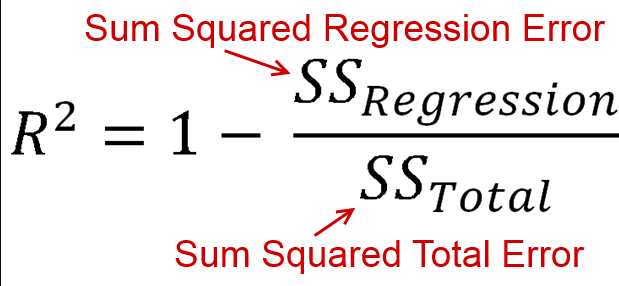top of page
• rishabhdwivedi062

# Model evaluation metrics in machine learning

Updated: Oct 5, 2022

They are used to calculate the errors in machine learning models, we will have a detailed look at various model evaluation techniques.

##### 1. Mean Error

This technique calculates the complete mean of the models at once. But the twist is that it will come out to be zero maximum time because some of the values are positive and some are negative hence overall it will be zero.

To overcome we will take absolute values.

##### 2. Mean Absolute Error (MAE)

This technique takes the absolute value of each individual error term and sums them up for all the data points, and then it takes its mean value

Hence it is the average of all the absolute errors.• n = the number of errors,

• Σ = summation symbol (which means “add them all up”),

• |xi – x| = the absolute errors.

3. Mean Square Error (MSE)

The concept of MSE is the same as that of MAE but they have greater penalties for higher values.##### 3. Root Mean Square Error (RMSE)

It gives the expected average distance between the actual points and the prediction. Hence it is the square root of MSE.If MAE is zero then it means the model is 100% accurate.

But if MAE = 250,00 then what does it means ???

To know this we need to understand the concept of R2 (R- Square)

##### R2

It gives us the relative error of a regression model with respect to the simple mean regression model.Hence we can say that SS(Regression) is equal to N*(MSE of the regression model we want to evaluate)

SS(Total) is equal to N*(MSE of the simple mean model)If R2 is 1 or 100% then it means it is a perfect model.

0<R2<1, hence its value lies between 0 and 1, but in reality, it is between -infinity to 1

The degree to which your input variable explains the variation of your target or output variable is known as R2.

If R2 = 0.8

It means 80% of the variable in the output variable is explained by the input variable.

The higher the R2 is, the more is the variation explained by the input variable, and hence better is the model.

The value of simple R2 can no longer be relied upon, as more input variables are added to the regression model.

The best linear regression model is the one that has the least errors.

You need not to learn all those formulas, sklearn python has everything already implemented, we will see it in the coming posts, till then keep learning.)